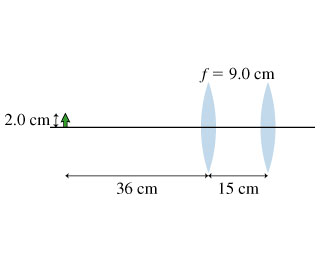# Problem: The figure shows a combination of two identical lenses. (Intro 1 figure)A. Find the position of the final image of the 2.0-cm-tall object.B. Find the size of the final image of the 2.0-cm-tall object.C. Find the orientation of the final image of the 2.0-cm-tall object.A. erectB. inverted

🤓 Based on our data, we think this question is relevant for Professor Sullivan's class at DIXIE.

###### FREE Expert Solution

Lens equation:
$\overline{)\frac{\mathbf{1}}{{\mathbit{s}}_{\mathbit{o}}}{\mathbf{+}}\frac{\mathbf{1}}{{\mathbit{s}}_{\mathbit{i}}}{\mathbf{=}}\frac{\mathbf{1}}{\mathbit{f}}}$

Magnification:

$\overline{){\mathbf{m}}{\mathbf{=}}\frac{{\mathbf{h}}_{\mathbf{i}}}{{\mathbf{h}}_{\mathbf{o}}}{\mathbf{=}}{\mathbf{-}}\frac{{\mathbf{s}}_{\mathbf{i}}}{{\mathbf{s}}_{\mathbf{o}}}}$

A.

We first determine the position of the image from the first lens.

1/si1 = 1/f - 1/so1

1/si1 = 1/9 - 1/36 = 1/12

si1 = 12 cm###### Problem Details

The figure shows a combination of two identical lenses. (Intro 1 figure)A. Find the position of the final image of the 2.0-cm-tall object.

B. Find the size of the final image of the 2.0-cm-tall object.

C. Find the orientation of the final image of the 2.0-cm-tall object.

A. erect

B. inverted